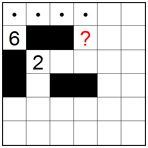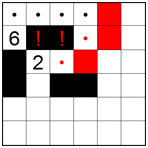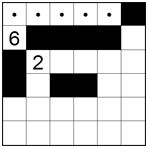﻿ Nurikabe решение
 ВходРегистрация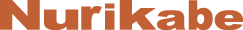# Nurikabe решение

Nurikabe is an island-forming puzzle based on a rectangular grid with clues in various places. The object is to create islands by partitioning between clues with walls so that each island contains exactly one clue and the number of squares in each island equals the value of the clue. In addition, all islands are isolated from each other horizontally and vertically, there are no wall areas of 2x2 or larger, and all walls form a continuous path when the puzzle is completed. In the example below we have a 6x6 Nurikabe puzzle with six columns and six rows. We now need to surround the clues with walls according to the above rules… but how?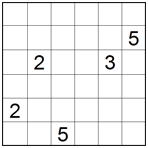## Starting techniques

Nurikabe puzzles frequently start off with some very easy moves between nearby clues. After solving the first moves, proceed to basic techniques to get more ideas how to continue solving the puzzles. Here are some ways of using the starting techniques:

#### 1. Island of 1

Since this is an island with a single square we can surround it with walls by shading the horizontally and vertically adjacent squares.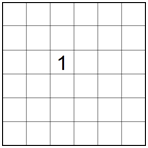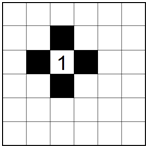#### 2. Clues separated by one square

According to Nurikabe rules, all clues must be partitioned from each other with walls. Therefore when two clues are in the same row or column and separated by one square, the square in between must be a wall.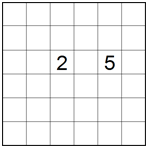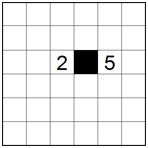#### 3. Diagonally adjacent clues

Similar to the example above, when two clues are diagonally adjacent then each of the squares touching both clues must be part of a wall.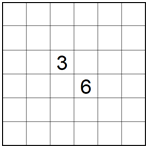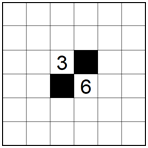## Basic techniques

We now continue with the basic techniques, where it is usually easy to see which squares are part of a wall and should be shaded, and which squares are part of an island and should be marked with a dot. Here are some ways of using the basic techniques:

#### 1. Surrounded square

After solving the first steps using the starting techniques described above, the puzzle in the left diagram below has two surrounded squares highlighted in red. Since these squares are surrounded by walls horizontally and vertically they cannot belong to an island and must therefore be shaded to be part of a wall.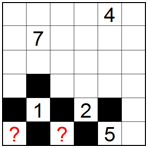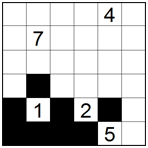#### 2. Wall expansion

According to Nurikabe rules, all walls must form a single continuous path. Looking at the single-square wall in the left diagram below, the only way to make it connect with the other walls is by expanding it with three squares on its right as shown in the right diagram.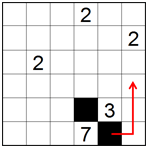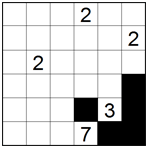#### 3. Wall continuity

In the puzzle below, if the highlighted square is part of an island then the wall on the top will become partitioned. Therefore, to maintain wall continuity according to Nurikabe rules, this square must be part of a wall.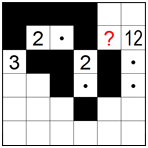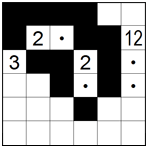#### 4. Island expansion from a clue

In many cases it is clear how an island can be expanded directly from a clue. In the diagram below, the island of 3 can only be expanded upwards and the island of 7 can only be expanded leftwards. We will mark these squares with dots to show they are part of the respective islands and cannot be part of a wall.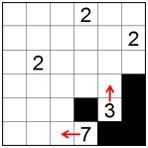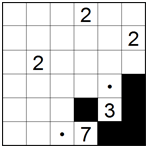#### 5. Island expandable only in two directions

In some cases an island of 2 or the last square of a larger island can only be expanded in two perpendicular directions. When this happens, regardless in which direction the island expansion will take place, the diagonal square must be part of a wall and is therefore shaded as shown in the right diagram below.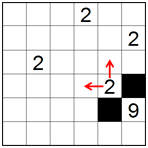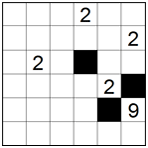#### 6. Hidden island expansion

Sometimes an island is too large to fit in a given area. In the partially solved puzzle below, the island of 12 cannot fit in the area at the top and must therefore expand downwards as marked with a dot in the diagram on the right. Note that now the square to the left of this dot cannot belong to any island and is therefore shaded to be part of a wall.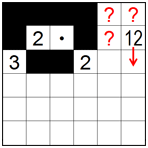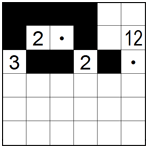#### 7. Island continuity

The square marked with a red dot in the left diagram below must be part of an island to avoid a wall area of 2x2. This means the square to its left must also be part of the same island and is therefore also marked with a dot. The island of 3 is now completed and we can surround it with a wall.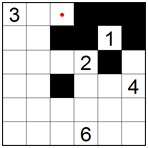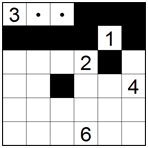#### 8. Surrounding a completed island

In the partially solved puzzle below, the island of 3 has been completed as shown in the highlighted area. We can now surround this island with horizontally and vertically adjacent walls as shown in the right image.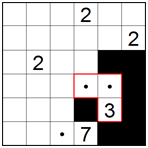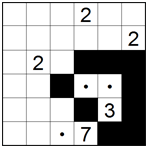#### 9. Avoiding wall area of 2x2

According to the rules it is not allowed to have wall areas of 2x2 or larger. Therefore the highlighted square below cannot be part of the wall and is marked with a dot showing it belongs to one of the islands.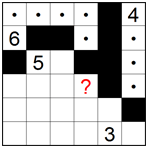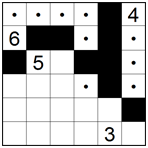#### 10. Unreachable square

In some cases a square cannot belong to any island simply because no clue can “reach” it. In the example below the two highlighted squares are too far from the clues and cannot be part of any island. They are therefore shaded to indicate they must be part of a wall.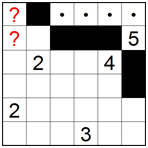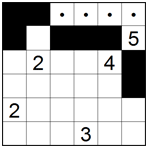The techniques described so far won’t be enough to solve hard puzzles. For this you will need advanced techniques to work out many special and interesting logic situations. Most advanced techniques use recursion, a looking ahead process of making assumptions and checking for conflicts one or two steps ahead. Here are some examples of advanced techniques to solve special situations. You will develop many more of your own when solving hard Nurikabe puzzles by yourself:

#### 1. Advanced technique 1

The highlighted square under the 2 in the left diagram below, like any other square in Nurikabe, can be either an island or a wall. Let’s assume it is part of an island, which means the island of 2 can be completed and surrounded with walls as shown in the center diagram. However, this will create a 2x2 wall area which is not allowed. Therefore the square under the 2 must be part of a wall as shown in the diagram on the right.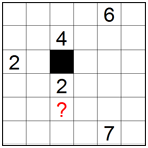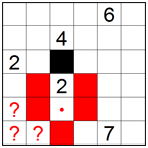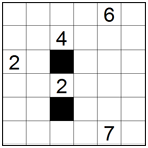#### 2. Advanced technique 2

Similar to the previous example, if we assume the highlighted square is part of a wall then once again a 2x2 wall area will be created which is not allowed. Therefore the highlighted square must be part of an island and is marked with a dot as shown in the diagram on the right.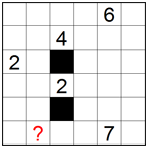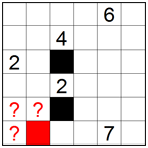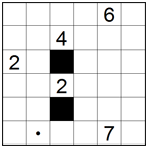#### 3. Advanced technique 3

If the highlighted square in the left diagram below is part of an island then the square under the 2 must be part of a wall. However, this part of a wall will not be able to connect with any other walls in the puzzle as shown in the center diagram. The highlighted square must therefore be part of a wall and is shaded as shown in the diagram on the right.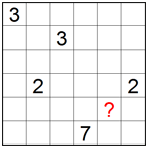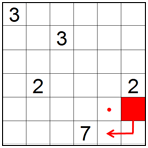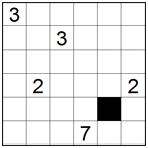#### 4. Advanced technique 4

Let’s look at the highlighted square in the partially solved puzzle shown in the left diagram below. If this square is part of a wall then both adjacent squares become unreachable and must be part of the wall too thus creating a 2x2 wall area. Since according to the rules of Nurikabe this is not allowed, the highlighted square must be part of an island and is marked with a dot in the diagram on the right.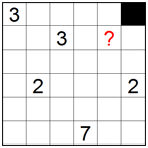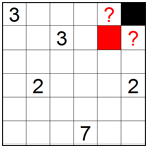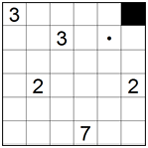#### 5. Advanced technique 5

Below is a section of a larger partially solved puzzle in which the island of 6 needs one last square to be completed. If we assume this square is the one highlighted in red in the left diagram below then the square under it must be part of a wall and the square on the left of this wall must be part of an island to avoid a 2x2 wall area. All this, as shown in the center diagram, will completely surround the wall on the right of the 6 eliminating wall continuity in all directions. Therefore the last square of the island of 6 must be towards the right and this island can be completed as shown in the diagram on the right.